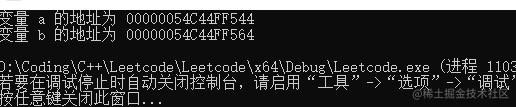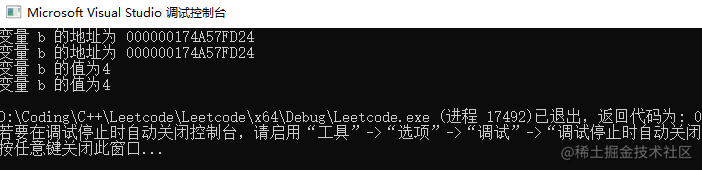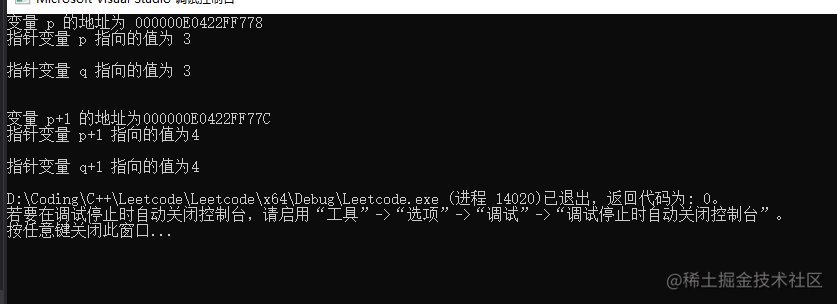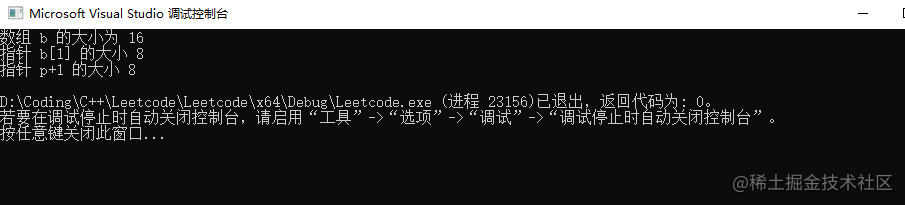# C++ 指针详讲、及指针与数组

• 信息存储在何处，也就是地址；
• 存储的值为多少，简称值；
• 存储的信息是什么类型，简称类型；

1，地址运算符&

``````int main()
{
int a = 3;
int b = 4;

cout << "变量 a 的地址为 " << (&a) << endl;
cout << "变量 b 的地址为 " << (&b) << endl;

return 0;
}2，指针的定义和用法

• 变量地址可用两种方式表示，p 和 &b;

• 变量值也同样由两种方式表示，*p 和 b;

``````int main()
{
int* p;//定义一个指针p 类型为 int;
int b = 4;

p = &b;//将 p 指向 b;

cout << "变量 b 的地址为 " << p << endl;
cout << "变量 b 的地址为 " << (&b) << endl;
cout << "变量 b 的值为" << b << endl;
cout << "变量 b 的值为" << *p << endl; // *p 表示指针p中存储的值；

return 0;
}``````typename* p;// typename 表示类型，p表示变量；

``````int a =4;
int *pt =&a;

``````int *pt;//定义一个指向 int 类型的指针 pt;
*pt = 23;// 错误，指针 pt 未指向任何地址，

3，指针和数组

• 正常整数变量加 1，则值加 1；
• 指针变量加 1 则即等于原来的地址值加指向对象所占用的总字节数(比如 double 类型加一需要增加 8 个字节)，根据指向指向地址的变化，*p(指针指向的值) 也同样发生变化

• 将一个数组名 arr 赋给一个指针 p 时 `int *p = arr`(类型是一致的) ，则代表指针 p 指向该数组第一个元素所在的地址 arr `p = arr`
• 当指针加1时，而指针指向的值为数组原来索引加 1 后所在的值 `*(p+1) == arr[n+1]`

``````int main()
{
int* p;//定义一个指针p 类型为 int;
int *q;
int b = {3,4};

p = &b;//将 p 指向 b;
q = b;// 指针 q 指向数组 b 的第一个元素

cout << "变量 p 的地址为 " << p << endl;
cout << "指针变量 p 指向的值为 " << *p << endl;
cout << endl;
cout << "指针变量 q 指向的值为 " << *q << endl;

cout << endl;
cout << endl;
cout << "变量 p+1 的地址为" << p+1 << endl;
cout << "指针变量 p+1 指向的值为" << *(p+1) << endl; // *p 表示指针p中存储的值；
cout << endl;
cout << "指针变量 q+1 指向的值为" << *(q + 1) << endl; // *p 表示指针p中存储的值；

return 0;
}array 为数组时，array + n 表示的是一个指向数组索引为 n-1 的指针，用 sizeof(array +n)指向指针类型大小；

``````int main()
{
int* p;//定义一个指针p 类型为 int;
int *q;
int b = {3,4,5,6};

p = &b;//将 p 指向 b;
q = b;// 指针 q 指向数组 b 的第一个元素

cout << "数组 b 的大小为 " << sizeof(b) << endl;
cout << "指针 b 的大小 " << sizeof(b+1) << endl;
cout << "指针 p+1 的大小 " << sizeof(p+1) << endl;

return 0;
}